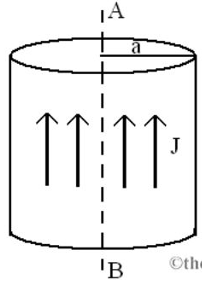# Problem: An electric current is flowing through a long cylindrical conductor with radius a = 0.15 m. The current density J= 5.5 A/m2 uniform in the cylinder. In this problem, we consider an imaginary cylinder with radius r around the axis AB (a) When r is less than a, express the current inside the imaginary cylinder ir in terms of r and J.(b) Express the magnitude of the magnetic field B at r in terms of the current through the imaginary cylinder ir and its radius.

###### FREE Expert Solution

The electric current in a conductor:

Ampere's law:

(a)

At r less than a, the current in the conductor is:

ir = πr2

83% (431 ratings)###### Problem Details

An electric current is flowing through a long cylindrical conductor with radius a = 0.15 m. The current density J= 5.5 A/m2 uniform in the cylinder. In this problem, we consider an imaginary cylinder with radius r around the axis AB

(a) When r is less than a, express the current inside the imaginary cylinder ir in terms of r and J.

(b) Express the magnitude of the magnetic field B at r in terms of the current through the imaginary cylinder ir and its radius.